# Image Normalization Using External Memory

This example shows how to normalize image pixel values using external memory. The example includes two models that show two ways to model the external memory: SoC external memory modeling and behavioral memory modeling. The example also verifies that the results of the two memory models are the same.

### Supported Hardware Platform

• Xilinx® Zynq® ZC706 evaluation kit for the ImageNormalizationHDLExample model

• Xilinx® Zynq® ZC706 evaluation kit and FMC-HDMI-CAM mezzanine card for the soc_imageNormalization_top model

### Introduction

The image normalization algorithm is a preprocessing step in deployment of deep learning networks on FPGA. This example provides an environment to prototype, customize, and integrate an end-to-end application in Simulink®, including a framework for memory-based system integration. The normalization algorithm that is implemented in this example takes reference from the rescale function.

In both models, the image normalization algorithm has these inputs and parameters.

• Input image: The image must be in RGB format, with pixels of uint8 data type.

• Lower bound and upper bound: These values are the range of the normalized output values. These values must be scalars in the range 0 to 255.

• Input minimum and maximum: These values are the minimum and maximum of the input pixel values. You can provide these parameters on the subsystem mask, or you can select the Compute input minimum and maximum parameter to automatically calculate these values.

This figure shows the subsystem mask parameters when you clear the Compute input minimum and maximum parameter and use fixed values for the Input minimum and Input maximum parameters.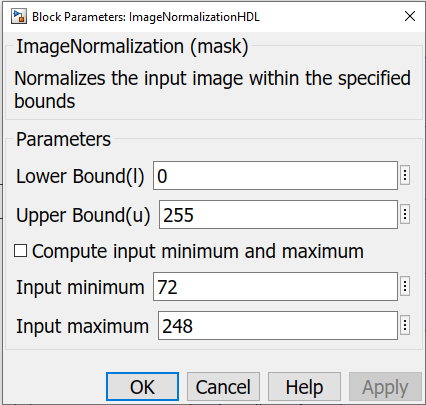This figure shows the subsystem mask parameters when you select the Compute input minimum and maximum parameter. The subsystem computes the input minimum and maximum values from the input pixel stream.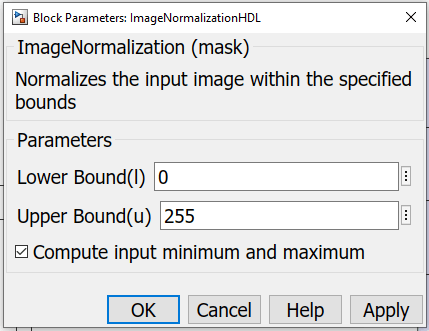To dynamically calculate the input minimum and maximum of the input frame, the design must store a complete frame in memory. This example shows two ways to model the frame memory. The ImageNormalizationHDLExample model stores the input frame by using HDL Coder™ FIFO blocks as a behavioral memory model. The soc_imageNormalization_top model stores the input frame by using SoC Blockset™ external memory blocks. Using external memory reduces the use of BRAM and enables processing of higher resolution input video streams. The use of external memory requires using AXI4 protocols and verification against memory contention. The model shows a fully compliant AXI4 interface that includes AXI4 write and read controllers.

The AXI4 random access interface provides a simple, direct interface to the memory interconnect. This protocol enables the algorithm to act as a memory controller by providing the addresses and managing the burst transfer directly. The AXI4-Master Write Controller and AXI4-Master Read Controller blocks in this example model a simplified AXI4 interface in Simulink™. When you generate HDL code using the HDL Coder product, the generated code includes a fully compliant AXI4 interface IP.

### External Memory Model

The SoC Blockset product provides Simulink blocks and visualization tools for modeling, simulating, and analyzing hardware and software architectures for ASICs, FPGAs, and SoCs. The product enables you to build a system architecture using memory models, bus models, and I/O models, and to simulate the architecture together with the algorithms. This example models the external memory interface by using the SoC Blockset Memory Channel and Memory Controller blocks.

• The Memory Channel block streams data through external memory. It models data transfer between the read and write controller algorithms, through shared memory. This example configures the channel to use AXI4 random access protocol.

• The Memory Controller block arbitrates between channels and grants them unique access to shared memory. It supports multiple channels with various arbitration protocols. The Memory Controller block also logs and displays performance data. This data enables you to debug and observe the performance of the system at simulation time.

### HDL Implementation

This figure shows the top level of the soc_imageNormalization_top model, including the Memory Channel and Memory Controller blocks. The HDMI Rx block processes the video input and passes it to the soc_imageNormalization_FPGA reference model.

open_system('soc_imageNormalization_top')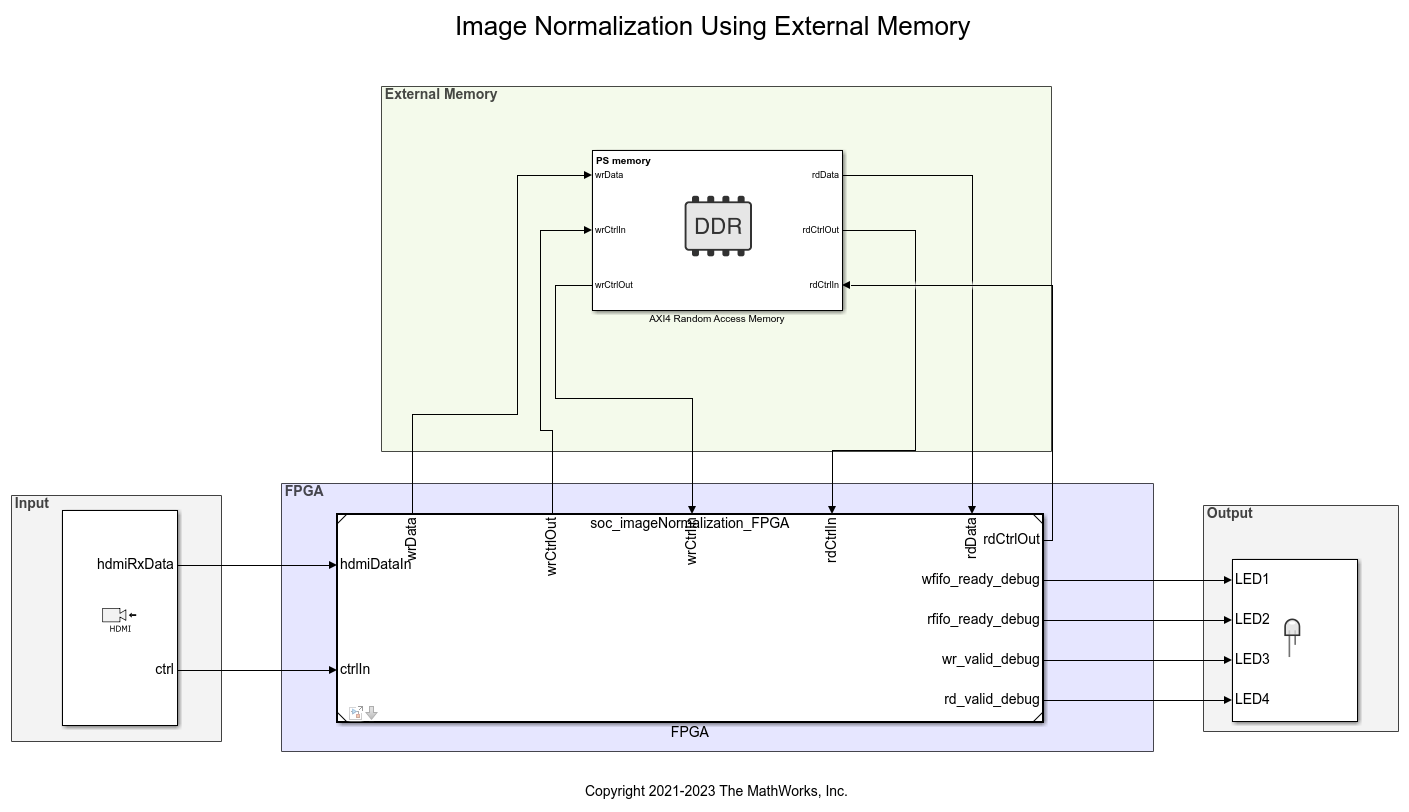In the soc_imageNormalization_FPGA model, the input pixel stream connects to a Video Stream Connector block. This block provides a video streaming interface to connect any two IPs in the FPGA implementation. The Video Stream Connector blocks connect the HDMI input and output blocks with the rest of the FPGA algorithm.

open_system('soc_imageNormalization_FPGA')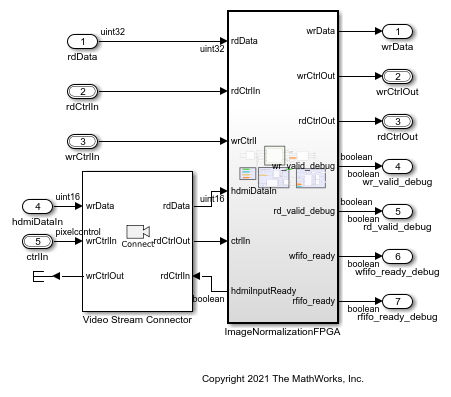The next figure shows the ImageNormalizationFPGA subsystem, which implements the AXI write and read from external memory and the normalization algorithm.

The hdmiDataIn signal is in YCbCr 4:2:2 pixel stream format. Because the normalization algorithm expects RGB images, the YCbCr422ToRGB subsystem converts the YCbCr 4:2:2 data to RGB.

The subsystem contains the ImageNormalization subsystem and these sections.

• AXI Write to Memory: This section writes the input data into the memory. It consists of an AXI4-Master Write Controller block that receives the input video control information from the HDMI Rx block and models the AXI4 memory-mapped interface for writing data into the DDR. It has five output signals: wr_addr, wr_len, wr_valid, rd_start, and frame. The wr_valid signal is an input to the AXI Write FIFO block, which stores the incoming pixel intensities. The SoC Bus Creator block generates the wrCtrlOut bus for writing the data into the DDR. The model writes one line of data per burst. After writing all of the lines of the frame, the model asserts the rd_start signal to begin the read request.

• AXI Read from Memory: This section reads the data from the memory. It consists of an AXI4-Master Read Controller block that receives the rd_start signal from the AXI4-Master Write Controller block. The AXI4-Master Read Controller block generates the rd_addr, rd_len, rd_avalid, and rd_dready signals. An SoC Bus Creator block combines these signals into a bus. The AXI4-Master Read Controller block also generates the pixelcontrol bus corresponding to the rd_data signal. The model slices the 32 bit rd_data signal to retrieve the 24 bit (LSB) RGB data. Then, the model forms a 1-by-3 uint8 RGB vector and passes the vector to the normalization algorithm.

The RGB pixel values read from the DDR frame memory are connected to the buffPixIn and buffCtrlIn input ports of the Image Normalization subsystem.

open_system('soc_imageNormalization_FPGA/ImageNormalizationFPGA')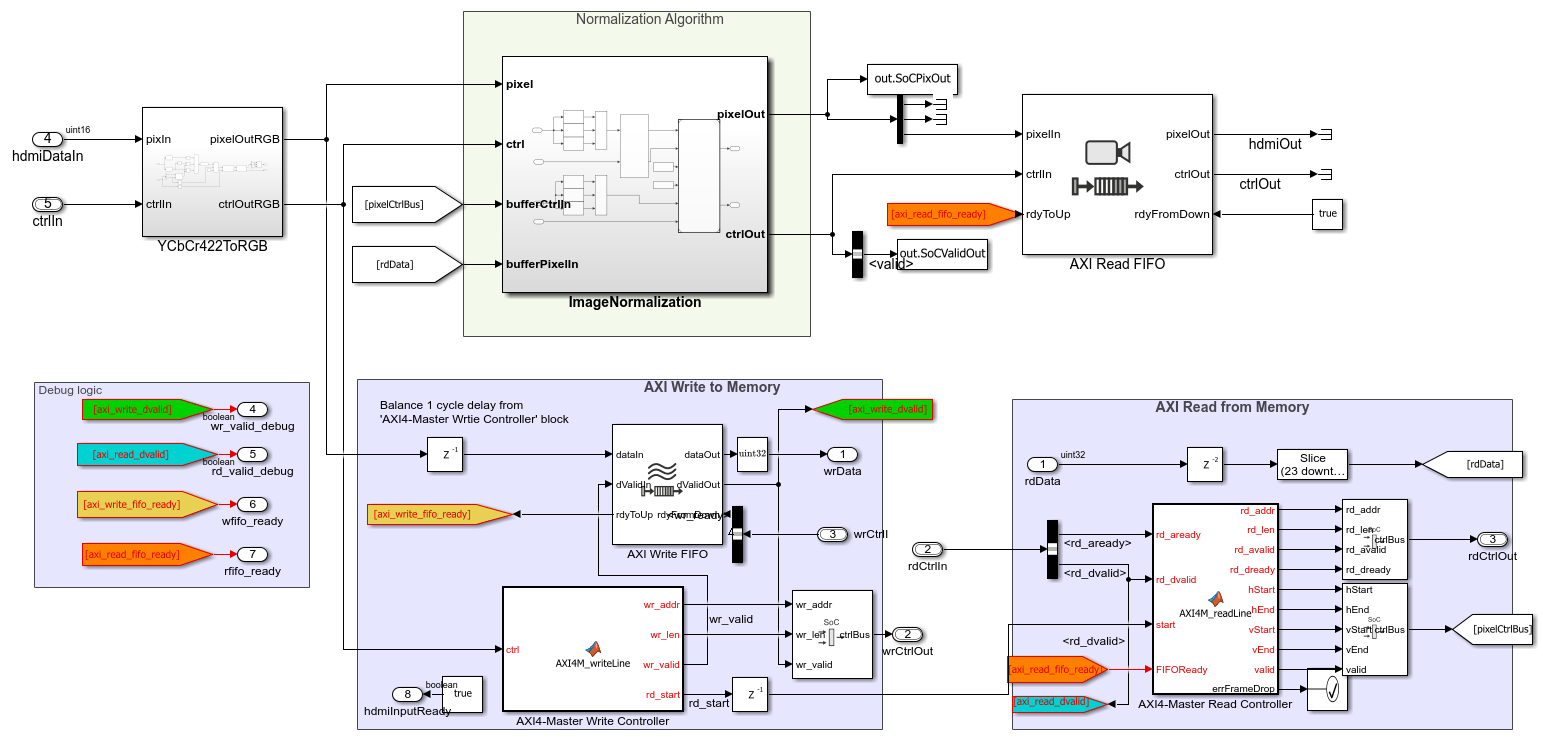### Normalization Algorithm

The next figure shows the ImageNormalization subsystem, which implements the normalization algorithm.

The input RGB pixel data (from the YCbCr422ToRGB subsystem) is of ufix24 data type. This subsystem converts the RGB data to uint8 1-by-3 RGB vectors. The InputMinMaxCalc subsystem calculates the input minimum and maximum values.

The Rescale subsystem references the NormalizationAlgorithm model.

open_system('soc_imageNormalization_FPGA/ImageNormalizationFPGA/ImageNormalization')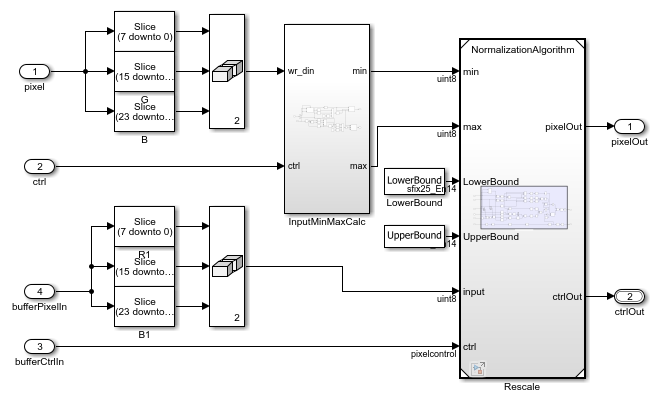The NormalizationAlgorithm model performs the normalization algorithm described by this equation.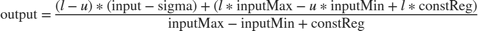l is the lower bound, u is the upper bound, sigma is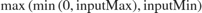, and constReg is high when the input minimum is equal to the input maximum.

This figure shows the NormalizationAlgorithm model.

open_system('NormalizationAlgorithm')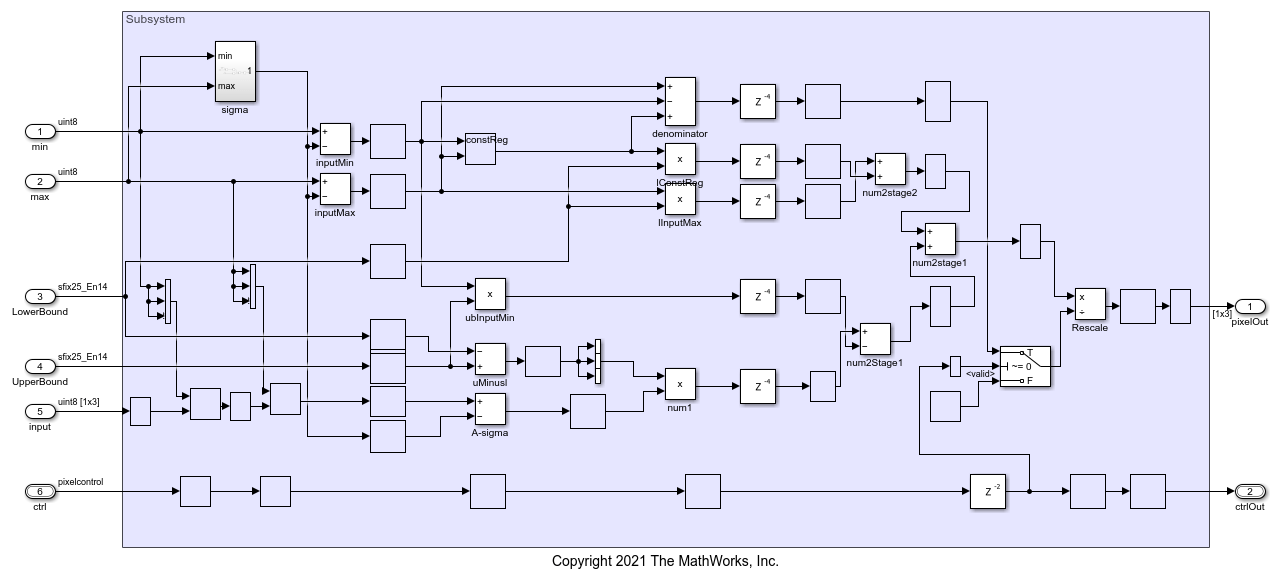### Hardware Implementation

To build, load, and execute the model on FPGA boards, use the SoC Builder tool. This example uses the Xilinx Zynq ZC706 evaluation kit. For more detail about the building steps, see SoC Builder (SoC Blockset).

### Performance Plots

This example uses an input video of size 480-by-640 pixels. The model configures the HDMI Rx block to use this size. For the Xilinx Zynq ZC706 evaluation kit, the PL DDR controller is configured with a 64 bit AXI4 subordinate interface running at 200 MHz. The resulting bandwidth is 1600 MB/s. This example has two AXI managers connected to the DDR controller. These AXI managers are the AXI4 read and write interfaces of the normalization algorithm. The YCbCr 4:2:2 video format requires 2 bytes per pixel. For the AXI4 read and write interfaces, each pixel is zero-padded to 4 bytes. In this case, the read and write interfaces have a throughput requirement of 2x4x480x640x60 = 147.456 MB/s.

This figure shows the performance plot of the Memory Controller block. To view the performance plot, first open the Memory Controller block. Then, on the Performance tab, click View performance plots. Select all of the masters under Bandwidth, and then click Update. After the algorithm starts writing and reading data into external memory, the throughput remains around 180 MB/s, which is within the required throughput of 147.456 MB/s.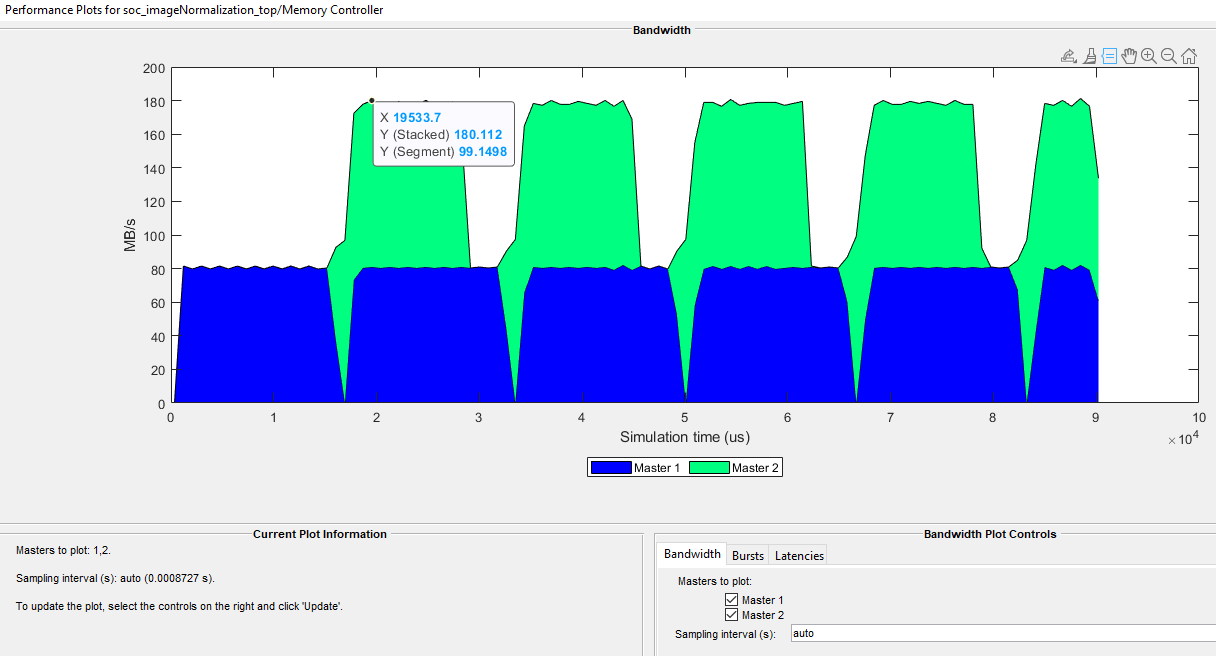### Behavioral Memory Model

This model implements the algorithm using a streaming pixel format, Vision HDL Toolbox™ blocks, and Simulink blocks that support HDL code generation. The serial interface mimics a real-time system and is efficient for hardware designs because less memory is required to store pixel data for computation. The serial interface also enables the design to operate independently of image size and format and makes the design more resilient to timing errors. Fixed-point data types use fewer resources and can give better performance on FPGA. The InitFcn callback function initializes the necessary variables for this example.

open_system('ImageNormalizationHDLExample');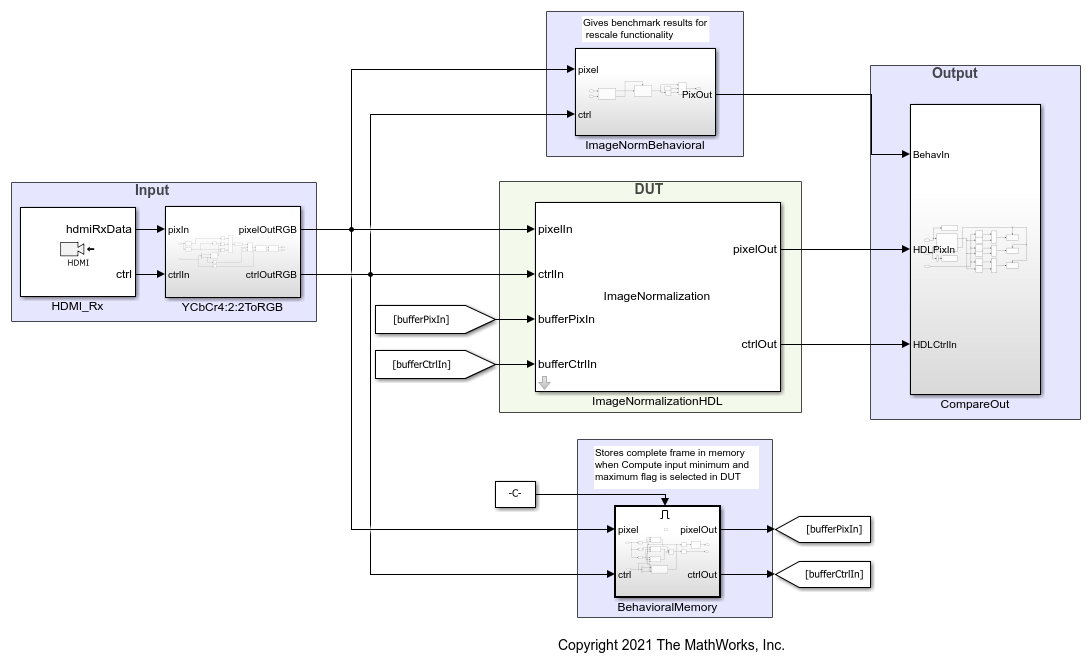The HDMI_Rx block imports the input video to the model. The Pixels To Frame block converts the pixel stream back to image frames. The BehavioralMemory subsystem stores the input image so that the NormalizationAlgorithm subsystem can read it as needed.

The ImageNormalizationHDL subsystem is a variant subsystem that provides either of the two implementations shown in this figure.

open_system('ImageNormalizationHDLExample/ImageNormalizationHDL/Variant Subsystem')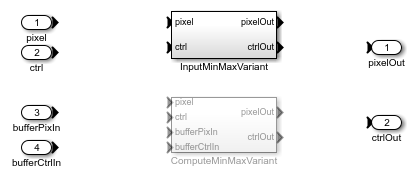### InputMinMaxVariant

If you clear the Compute input minimum and maximum parameter, then you must provide Input minimum and Input maximum parameter values. The algorithm normalizes the input frame by using the provided input minimum and maximum values and the lower and upper bound values.

open_system('ImageNormalizationHDLExample/ImageNormalizationHDL/Variant Subsystem/InputMinMaxVariant')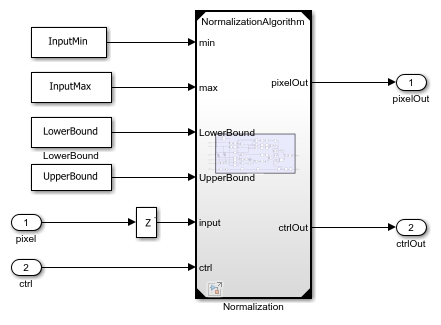### ComputeMinMaxVariant

If you select the Compute input minimum and maximum parameter, then the InputMinMaxCalc subsystem computes the input minimum and maximum values of the input image. The algorithm normalizes the input frame by using the computed input minimum and maximum values and the provided lower and upper bound values.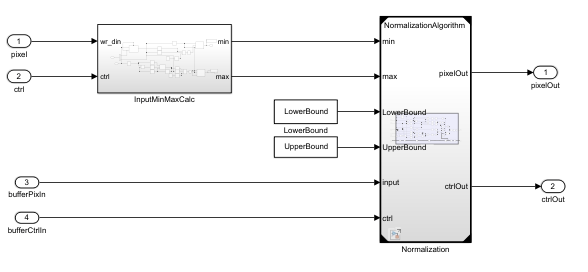You can verify the results from either of the variant implementations against the golden reference normalization algorithm by using the CompareOut block.

open_system('ImageNormalizationHDLExample/CompareOut')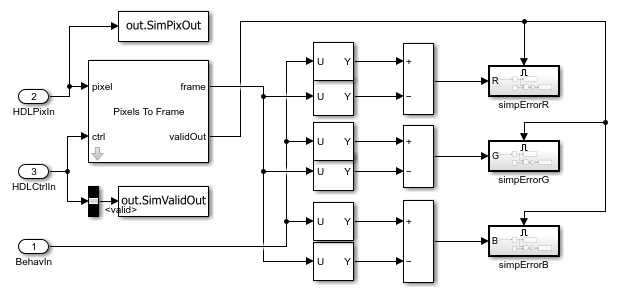### Verify Results Between External Memory Model and Behavioral Memory Model

Compare the output from the ImageNormalizationHDLExample model (behavioral memory model) with the output of the soc_imageNormalization_top model (external memory model) by using the errorCheck.m script. To be able to compare the results of these two models, you must select the Compute input minimum and maximum parameter in the ImageNormalizationHDLExample model. Run both models to save the output to the MATLAB® workspace. The outputs of the ImageNormalizationHDLExample model are the simPixOut and simValidOut variables. The outputs of the soc_imageNormalization_top model are the socPixOut and socValidOut variables. The errorCheck function takes these variables as inputs and returns the total number of error pixels in the R, G, and B channels.

 [errR,errG,errB] = errorCheck(simPixOut,simValidOut,socPixOut,socValidOut)